# The most confusing notation in number theory

This is an observation in abstractmath that I think needs to be publicized more:

Two symbols used in the study of integers are notorious for their confusing similarity.

• The expression “$m/n$” is a term denoting the number obtained by dividing $m$ by $n$. Thus “$12/3$” denotes $4$ and “$12/5$” denotes the number $2.4$.
• The expression “$m|n$” is the assertion that “$m$ divides $n$ with no remainder”. So for example “$3|12$”, read “$3$ divides $12$” or “$12$ is a multiple of $3$”, is a true statement and “$5|12$” is a false statement.

Notice that $m/n$ is an integer if and only if $n|m$. Not only is $m/n$ a number and $n|m$ a statement, but the statement “the first one is an integer if and only if the second one is true” is correct only after the $m$ and $n$ are switched!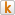Send to Kindle

# Definition of function

Note: This is a revision of the article on specification and definition of functions from abstractmath.org. Many of the links in this article take you to other articles in abstractmath.org.

 A function is a mathematical object.

To deal with functions as a math object, you need a precise definition of “function”. That is what this article gives you.

• The article starts by giving a specification of “function”.
• After that, we get into the technicalities of the
definitions of the general concept of function.
• Things get complicated because there are several inequivalent definitions of “function” in common use.

## Specification of “function”

A function $f$ is a mathematical object which determines and is completely determined by the following data:

• (DOM) $f$ has a domain, which is a set. The domain may be denoted by $\text{dom} f$.
• (COD) $f$ has a codomain, which is also a set and may be denoted by $\text{cod} f$.
• (VAL) For each element $a$ of the domain of $f$, $f$ has a value at $a$.
• (FP) The value of $f$ at $a$ is
completely determined by $a$ and $f$.
• (VIC) The value of $f$ at $a$ must be an element of the codomain of $f$.

• The value of $f$ at $a$ is most cohttps://abstractmath.org/MM/MMonly written $f(a)$, but see Functions: Notation and Terminology.
• To evaluate $f$ at $a$ means to determine $f(a)$. The two examples of functions below show that different functions may have different strategies for evaluating them.
• In the expression “$f(a)$”, $a$ is called the input or (old-fashioned) argument of $f$.
• “FP” means functional property.
• “VIC” means “value in codomain”.

### Examples

I give two examples here. The examples of functions chapter contains many other examples.

#### A finite function

Let $F$ be the function defined on the set $\left\{\text{a},\text{b},\text{c},\text{d}\right\}$ as follows: $F(\text{a})=\text{a},\,\,\,F(\text{b})=\text{c},\,\,\,F(\text{c})=\text{c},\,\,\,F(\text{d})=\text{b}$In this definition, $\text{a},\text{b},\text{c},\text{d}$ are letters of the alphabet, not variables. This is the function called “Finite” in the chapter on examples of functions.

• The definition of $F$ says “$F$ is defined on the set $\left\{\text{a},\,\text{b},\,\text{c},\,\text{d} \right\}$”. The phrase “is defined on”
means that the domain is that set. That is standard terminology.
• The value of $F$ at each element of the domain is given explicitly. The value at
$\text{b}$, for example, is $\text{c}$, because the definition says that $F(\text{b}) = \text{c}$. No other reason needs to be given. Mathematical definitions can be arbitrary.
• The codomain of $F$ is not specified, but must include the set $\{\text{a},\text{b},\text{c}\}$. The codomain of a function is often not specified when it is not important, which is most of the time in freshman calculus (for example).
• The diagram below shows how $F$ obeys the rule that the value of an element $x$ in the domain is completely determined by $x$ and $F$.
•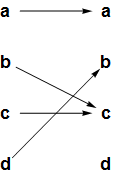• If two arrows had started from the same element of the domain, then $F$ would not be a function. (It would be a multivalued function).
• If there were an element of the domain that no arrow started from, it $F$ would not be a function. (It would be a partial function.)
• In this example, to evaluate $F$ at $b$ (to determine the value of $F$ at $b$) means to look at the definition of $F$, which says among other things that the value is $c$ (or alternatively, look at the diagram above and see what letter the arrow starting at $b$ points to). In this case, “evaluation” does not imply calculating a formula.

#### A real-valued function

Let $G$ be the real-valued function defined by the formula $G(x)={{x}^{2}}+2x+5$.

• The definition of $G$ gives the value at each element of the domain by a formula. The value at $3$, for example, is obtained by calculating $G(3)=3^2+2\cdot3+5=20$
• The definition of $G$
does not specify the domain. The convention in the case of functions defined on the real numbers by a formula is to take the domain to be all real numbers at which the formula is defined. In this case, that is every real number, so the domain is $\mathbb{R}$.
• The definition of $G$ does not specify the codomain, either. However, the codomain must include all real numbers greater than or equal to $4$. (Why?)
• So if an author wrote, “Let $H(x)=\frac{1}{x}$”, the domain would be the set of all real numbers except $0$. But a careful author would write, “Let $H(x)=\frac{1}{x}$ ($x\neq0$).”

### What the specification means

• The specification guarantees that a function satisfies all five of the properties listed.
• The specification does not define a mathematical structure in the way mathematical structures have been defined in the past: In particular, it does not require a function to be one or more sets with structure.
• Even so, it is useful to have the specification, because:

 Many mathematical definitions introduce extraneous technical elements which clutter up your thinking about the object they define.

## History

The discussion below is an over­simpli­fication of the history of mathe­matics, which many people have written thick books about. A book relevant to these ideas is Plato’s Ghost, by Jeremy Gray.

Until late in the nineteenth century, functions were usually thought of as defined by formulas (including infinite series). Problems arose in the theory of harmonic analysis which made mathematicians require a more general notion of function. They came up with the concept of function as a set of ordered pairs with the functional property (discussed below), and that understanding revolutionized our understanding of math.

In particular, this definition, along with the use of set theory, enabled abstract math (ahem) to become a cohttps://abstractmath.org/MM/MMon tool for understanding math and proving theorems. It is conceivable that some readers may wish it hadn’t. Well, tough.

The modern definition of function given here (which builds on the ordered pairs with functional property definition) came into use beginning in the 1950’s. The modern definition became necessary in algebraic topology and is widely used in many fields today.

The concept of function as a formula never disappeared entirely, but was studied mostly by logicians who generalized it to the study of function-as-algorithm. Of course, the study of algorithms is one of the central topics of modern computing science, so the notion of function-as-formula (updated to function-as-algorithm) has achieved a new importance in recent years.

To state both the definition, we need a preliminary idea.

## The functional property

A set $P$ of ordered pairs has the functional property if two pairs in $P$ with the same first coordinate have to have the same second coordinate (which means they are the same pair). In other words, if $(x,a)$ and $(x,b)$ are both in $P$, then $a=b$.

#### How to think about the functional property

The point of the functional property is that for any pair in the set of ordered pairs, the first coordinate determines what the second one is (which is just what requirement FP says in the specification). That’s why you can write “$G(x)$” for any $x$ in the domain of $G$ and not be ambiguous.

#### Examples

• The set $\{(1,2), (2,4), (3,2), (5,8)\}$ has the functional property, since no two different pairs have the same first coordinate. Note that there are two different pairs with the same second coordinate. This is irrelevant to the functional property.
• The set $\{(1,2), (2,4), (3,2), (2,8)\}$ does not have the functional property. There are two different pairs with first coordinate 2.
• The empty set $\emptyset$ has the function property vacuously.

#### Example: graph of a function defined by a formula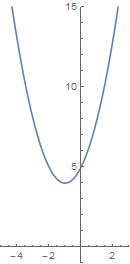In calculus books, a picture like this one (of part of $y=x^2+2x+5$) is called a graph. Here I use the word “graph” to denote the set of ordered pairs
$\left\{ (x,{{x}^{2}}+2x+5)\,\mathsf{|}\,x\in \mathbb{R } \right\}$
which is a mathematical object rather than some ink on a page or pixels on a screen.

The graph of any function studied in beginning calculus has the functional property. For example, the set of ordered pairs above has the functional property because if $x$ is any real number, the formula ${{x}^{2}}+2x+5$ defines a specific real number.

• if $x = 0$, then ${{x}^{2}}+2x+5=5$, so the pair $(0, 5)$ is an element of the graph of $G$. Each time you plug in $0$ in the formula you get 5.
• if $x = 1$, then ${{x}^{2}}+2x+5=8$.
• if $x = -2$, then ${{x}^{2}}+2x+5=5$.

You can measure where the point $\{-2,5\}$ is on the (picture of) the graph and see that it is on the blue curve as it should be. No other pair whose first coordinate is $-2$ is in the graph of $G$, only $(-2, 5)$. That is because when you plug $-2$ into the formula ${{x}^{2}}+2x+5$, you get $5$ and nothing else. Of course, $(0, 5)$ is in the graph, but that does not contradict the functional property. $(0, 5)$ and $(-2, 5)$ have the same second coordinate, but that is OK.

## Mathematical definition of function

A function $f$ is a
mathematical structure consisting of the following objects:

• A set called the domain of $f$, denoted by $\text{dom} f$.
• A set called the codomain of $f$, denoted by $\text{cod} f$.
• A set of ordered pairs called the graph of $f$, with the following properties:
• $\text{dom} f$ \text{dom} fis the set of all first coordinates of pairs in the graph of $f$.
• Every second coordinate of a pair in the graph of $f$ is in $\text{cod} f$ (but $\text{cod} f$ may contain other elements).
• The graph of $f$ has the functional property.

Using arrow notation, this implies that $f:\text{dom}f\to\text{cod} f$.

#### Remark

The main difference between the specification of function given previously and this definition is that the definition replaces the statement “$f$ has a value at $a$” by introducing a set of ordered pairs (the graph) with the functional property.

• This set of ordered pairs is extra structure introduced by the definition mainly in order to make the definition a classical sets-with-structure.
• This makes the graph, which should be a concept derived from the concept of function, appear to be a necessary part of the function.
• That suggests incorrectly that the graph is more of a primary intuition that other intuitions such as function as map, function as transformer, and other points of view discussed in the article Images and meta­phors for functions.
• The concept of graph of a function is indeed an important intuition, and is discussed with examples in the articles Graphs of continuous functions and Graphs of finite functions.
• Nevertheless, the fact that the concept of graph appears in the definition of function does not make it the most important intuition.

### Examples

• Let $F$ have graph $\{(1,2), (2,4), (3,2), (5,8)\}$ and define $A = \{1, 2, 3, 5\}$ and $B = \{2, 4, 8\}$. Then $F:A\to B$ is a function. In speaking, we would usually say, “$F$ is a function from $A$ to $B$.”
• Let $G$ have graph $\{(1,2), (2,4), (3,2), (5,8)\}$ (same as above), and define $A = \{1, 2, 3, 5\}$ and $C = \{2, 4, 8, 9, 11, \pi, 3/2\}$. Then $G:A\to C$ is a (admittedly ridiculous) function. Note that all the second coordinates of the graph are in the codomain $C$, along with a bunch of miscellaneous suspicious characters that are not second coordinates of pairs in the graph.
• Let $H$ have graph $\{(1,2), (2,4), (3,2), (5,8)\}$. Then $H:A\to \mathbb{R}$ is a function, since $2$, $4$ and $8$ are all real numbers.
• Let $D = \{1, 2, 5\}$ and $E = \{1, 2, 3, 4, 5\}$. Then there is no function $D\to A$ and no function $E\to A$ with graph $\{(1,2), (2,4), (3,2), (5,8)\}$. Neither $D$ nor $E$ has exactly the same elements as the first coordinates of the graph.

### Identity and inclusion

Suppose we have two sets  A and  B with $A\subseteq B$.

• The identity function on A is the function ${{\operatorname{id}}_{A}}:A\to A$ defined by ${{\operatorname{id}}_{A}}(x)=x$ for all $x\in A$. (Many authors call it ${{1}_{A}}$).
• When $A\subseteq B$, the inclusion function from $A$ to $B$ is the function $i:A\to B$ defined by $i(x)=x$ for all $x\in A$. Note that there is a different function for each pair of sets $A$ and $B$ for which $A\subseteq B$. Some authors call it ${{i}_{A,\,B}}$ or $\text{in}{{\text{c}}_{A,\,B}}$.

The identity function and an inclusion function for the same set $A$ have exactly the same graph, namely $\left\{ (a,a)|a\in A \right\}$. More about this below.

## Other definitions of function

### Original abstract definition of function

#### Definition

• A function $f$ is a set of ordered pairs with the functional property.
• If $f$ is a function according to this definition, the domain of $f$ is the set of first coordinates of all the pairs in $f$.
• If $x\in \text{dom} f$, then we define the value of $f$ at $x$, denoted by $f(x)$, to be the second coordinate of the only ordered pair in $f$ whose first coordinate is $x$.

#### Remarks

• This definition is still widely used in mathematical writing.
• Many authors do not tell you which definition they are using.
• For many purposes (including freshman calculus for the most part) it does not matter which definition is used.
• In some branches of math, the modern definition adds great clarity to many complicated situations; using the older definition can even make it difficult to describe some important constructions. There is more about this in New Approaches below.

#### Possible confusion

Some confusion can result because of the presence of these two different definitions.

• For example, since the identity function ${{\operatorname{id}}_{A}}:A\to A$ and the inclusion function ${{i}_{A,\,B}}:A\to B$ have the same graph, users of the older definition are required in theory to say they are the same function.
• Also it requires you to say that the graph of a function is the same thing as the function.
• In my observation, this does not make a problem in practice, unless there is a very picky person in the room.
• It also appears to me that the modern definition is (quite rightly) winning and the original abstract definition is disappearing.

### Multivalued function

The phrase multivalued function refers to an object that is like a function $f:S\to T$ except that for $s\in S$, $f(s)$ may denote more than one value.

#### Examples

• Multivalued functions arose in considering complex functions. In cohttps://abstractmath.org/MM/MMon practice, the symbol $\sqrt{4}$ denoted $2$, although $-2$ is also a square root of $4$. But in complex function theory, the square root function takes on both the values $2$ and $-2$. This is discussed in detail in Wikipedia.
• The antiderivative is an example of a multivalued operator. For any constant $C$, $\frac{x^3}{3}+C$ is an antiderivative of $x^2$, so that $\frac{x^3}{3}$, $\frac{x^3}{3}+42$, $\frac{x^3}{3}-1$ and $\frac{x^3}{3}+2\pi$ are among the infinitely many antiderivatives of $x^2$.

A multivalued function $f:S\to T$ can be modeled as a function with domain $S$ and codomain the set of all subsets of $T$. The two meanings are equivalent in a strong sense (naturally equivalent). Even so, it seems to me that they represent two differ­ent ways of thinking about
multivalued functions. (“The value may be any of these things…” as opposed to “The value is this whole set of things.”)

Some older mathematical papers in com­plex func­tion theory do not tell you that their functions are multi­valued. There was a time when com­plex func­tion theory was such a Big Deal in research mathe­matics that the phrase “func­tion theory” meant complex func­tion theory and every mathe­ma­tician with a Ph. D. knew that complex functions were multi­valued.

### Partial function

A partial function $f:S\to T$ is just like a function except that its input may be defined on only a subset of $S$. For example, the function $f(x):=\frac{1}{x}$ is a partial function from the real numbers to the real numbers.

This models the behavior of computer programs (algorithms): if you consider a program with one input and one output as a function, it may not be defined on some inputs because for them it runs forever (or gives an error message).

In some texts in computing science and mathematical logic, a function is by
convention a partial function, and this fact may not be mentioned explicitly, especially in research papers.

The phrases “multivalued function” and “partial function” upset some picky types who say things like, “But a multi­valued func­tion is not a func­tion!”. A hot dog is not a dog, either. I once had a Russian teacher who was Polish and a German teacher who was Hungarian. So what? See the Hand­book (click on

## New approaches to functions

All the definitions of function given here produce mathematical structures, using the traditional way to define mathematical objects in terms of sets. Such definitions have disadvantages.

Mathematicians have many ways to think about functions. That a function is a set of ordered pairs with a certain property (functional) and possibly some ancillary ideas (domain, codomain, and others) is not the way we usually think about them$\ldots$Except when we need to reduce the thing we are studying to its absolutely most abstract form to make sure our proofs are correct.
That most abstract form is what I have called the rigorous view or the dry bones and it is when that reasoning is needed that the sets-with-structure approach has succeeded.

Our practice of abstraction has led us to new approaches to talking about functions. The most important one currently is category theory. Roughly, a category is a bunch of objects together with some arrows going between them that can be composed head to tail. Functions between sets are examples of this: the sets are the objects and the functions the arrows. But arrows in a category do not have to be functions; in that way category theory is an abstraction of functions.

This abstracts the idea of function in a way that brings out common ideas in various branches of math. Research papers in many branches of mathematics now routinely use the language of category theory. Categories now appear in some undergraduate math courses, meaning that Someone needs to write a chapter on category theory for abstractmath.org.

Besides category theory, computing scientists have come up with other abstract ways of dealing with functions, for example type theory. It has not come as far along as category theory, but has shown recent signs of major progress.

Both category theory and type theory define math objects in terms of their effect on and relationship with other math objects. This makes it possible to do abstract math entirely without using sets-with-structure as a means of defining concepts.

## References

• Functions in Wikipedia. This is an extensive and mostly well-done description of the use of functions in mathematics.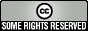Send to Kindle

# VARIABLE MATHEMATICAL OBJECTS

In many mathematical texts, the variable $x$ may denote a real number, although which real number may not be specified. This is an example of a variable mathematical object. This point of view and terminology is not widespread, but I think it is worth understanding because it provides a deeper understanding of some aspects about how math is done.

## Specific and variable mathematical objects

It is useful to distinguish between specific math objects and variable math objects.

#### Examples of specific math objects

• The number $42$ (the math object represented as “42” in base $10$, “2A” in hexadecimal and “XLII” as a Roman numeral) is a specific math object. It is an abstract math object. It is not any of the representations just listed — they are just strings of letters and numbers.
• The ordered pair $(4,3)$ is a specific math object. It is not the same as the ordered pair $(7,-2)$, which is another specific math object.
• The sine function $\sin:\mathbb{R}\to\mathbb{R}$ is a specific math object. You may know that the sine function is also defined for all complex numbers, which gives another specific math object $\sin:\mathbb{C}\to\mathbb{C}$.
• The group of symmetries of a square is a specific math object. (If you don’t know much about groups, the link gives a detailed description of this particular group.)

#### Variable math objects

Math books are full of references to math objects, typically named by a letter or a name, that are not completely specified. Some mathematicians call these variable objects (not standard terminology). The idea of a variable mathe­mati­cal object is not often taught as such in under­graduate classes but it is worth pondering. It has certainly clari­fied my thinking about expres­sions with variables.

#### Examples

• If an author or lecturer says “Let $x$ be a real variable”, you can then think of $x$ as a variable real number. In a proof you can’t assume that $x$ is any particular real number such as $42$ or $\pi$.
• If a lecturer says, “Let $(a,b)$ be an ordered pair of integers”, then all you know is that $a$ and $b$ are integers. This makes $(a,b)$ a variable ordered pair, specifically a pair of integers. The lecturer will not say it is a variable ordered pair since that terminology is not widely used. You have to understand that the phrase “Let $(a,b)$ be an ordered pair of integers” implies that it is a variable ordered pair just because “a” and “b” are letters instead of numbers.
• If you are going to prove a theorem about functions, you might begin, "Let $f$ be a continuous function", and in the proof refer to $f$ and various objects connected to $f$. This makes $f$ a variable mathematical object. When you are proving things about $f$ you may use the fact that it is continuous. But you cannot assume that it is (for example) the sine function or any other particular function.
• If someone says, “Let $G$ be a group” you can think of $G$ as a variable group. If you want to prove something about $G$ you are free to use the definition of “group” and any theorems you know of that apply to all groups, but you can’t assume that $G$ is any specific group.

#### Terminology

A logician would refer to the symbol $f$, thought of as denoting a function, as a vari­able, and likewise the symbol $G$, thought of as denoting a group. But mathe­maticians in general would not use the word “vari­able” in those situa­tions.

## How to think about variable objects

The idea that $x$ is a variable object means thinking of $x$ as a genuine mathematical object, but with limitations about what you can say or think about it. Specifically,

Some assertions about a variable math object
may be neither true nor false.

##### Example

The statement, “Let $x$ be a real number” means that $x$ is to be regarded as a variable real number (usually called a “real variable”). Then you know the following facts:

• The statement “${{x}^{2}}$ is not negative” is true.
• The assertion “$x=x+1$” is false.
• The assertion “$x\gt 0$” is neither true nor false.
##### Example

Suppose you are told that $x$ is a real number and that ${{x}^{2}}-5x=-6$.

• You know (because it is given) that the statement “${{x}^{2}}-5x=-6$” is true.
• By doing some algebra, you can discover that the statement “$x=2$ or $x=3$” is true.
• The statement “$x=2$ and $x=3$” is false, because $2\neq3$.
• The statement “$x=2$” is neither true nor false, and similarly for “$x=3$”.
• This situation could be described this way: $x$ is a variable real number varying over the set $\{2,3\}$.
##### Example

This example may not be easy to understand. It is intended to raise your consciousness.

A prime pair is an ordered pair of integers $(n,n+2)$ with the property that both $n$ and $n+2$ are prime numbers.

Definition: $S$ is a PP set if $S$ is a set of pairs of integers with the property that every pair is a prime pair.

• “$\{(3,5),(11,13)\}$ is a PP set” is true.
• “$\{(5,7),(111,113),(149,151)\}$ is a PP set” is false.

Now suppose $SS$ is a variable PP set.

• “$SS$ is a set” is true by definition.
• “$SS$ contains $(7,9)$” is false.
• “$SS$ contains $(3,5)$” is neither true nor false, as the examples just above show.
• “$SS$ is an infinite set”:
• This is certainly not true (see finite examples above).
• This claim may be neither true nor false, or it may be plain false, because no one knows whether there is an infinite number of prime pairs.
• The point of this example is to show that “we don’t know” doesn’t mean the same thing as “neither true nor false”.Send to Kindle

# Power

I have rewritten the entry to “power” in the abstractmath.org Glossary:

## POWER

Here are three variant phrases that say that $125=5^3$:

• “$125$ is a power of $5$ with exponent $3$”.
• “$125$ is the third power of $5$”.
• “$125$ is $5$ to the third power”.

Some students are confused by such statements, and conclude that $3$ is the “power”. This usage appears in print in Wikipedia in its entry on Exponentiation (as it was on 22 November 2016):

“…$b^n$ is the product of multiplying $n$ bases:

$b^n = \underbrace{b \times \cdots \times b}_n$

In that case, $b^n$ is called the $n$-th power of $b$, or $b$ raised to the power $n$.”

As a result, students (and many mathematicians) refer to $n$ as the “power” in any expression of the form “$a^n$”. The number $n$ should be called the “exponent”. The word “power” should refer only to the result $a^n$. I know mathematical terminology is pretty chaotic, but it is silly to refer both to $n$ and to $a^n$ as the “power”.

Almost as silly as using $(a,b)$ to refer to an open interval, an ordered pair and the GCD. (See The notation $(a,b)$.)

Suggestion for lexicographical research: How widespread does referring to $n$ as the “power” come up in math textbooks or papers? (See usage.)

Thanks to Tomaz Cedilnik for comments on the first version of this entry.Send to Kindle

# A slow introduction to category theory

Category theory turns math inside-out. Definitions depend on nothing inside, but on everything outside. — John Cook

This is a draft of the first part of an article on category theory that will be posted on abstractmath.org. It replaces an earlier version that was posted in June, 2016.

During the last year or so, I have been monitoring the category theory questions on Math Stack Exchange. Some of the queries are clearly from people who do not have enough of a mathematical background to understand basic abstract reasoning, for example the importance of definitions and the difficulties described in the abmath artice on Dysfunctional attitudes and behaviors. Category theory has become important in several fields outside mathematics, for example computer science and database theory.

This article is intended to get people started in category theory by giving a very detailed definition of “category” and some examples described in detail with an emphasis on how the example fits the definition of category. That’s all the present version does, but I intend to add some examples of constructions and properties such as the dual category, product, and other concepts that some of the inquirers on Math Stack Exchange had great difficulty with.

There is no way in which this article is a proper introduction to category theory. It is intended only to give beginners some help over the initial steps of understanding the subject, particularly the aspects of understanding that cause many hopeful math majors to fall off the Abstraction Cliff.

To be written.

# Definition of category

A category is a type of Mathematical structure consisting of two types of data, whose relationships are entirely determined by some axioms. After the definition is complete, I introduce several example categories with a detailed discussion of each one, explaining how they fit the definition of category.

## Axiom 1: Data

A category consists of two types of data: objects and arrows.

### Notes for Axiom 1

• You will see in the section on Examples of categories that every definition of a category $\mathsf{C}$ starts by specifying what the objects of $\mathsf{C}$ are and what the arrows of $\mathsf{C}$ are. That is what Axiom I requires.
• An object of a category can be any kind of mathematical object. It does not have to be a set and it does not have to have elements.
• Arrows of a category are also called morphisms. You may be familiar with “homomorphisms”, “homeomorphisms” or “isomorphisms”, all of which are functions. This does not mean that a “morphism” in an arbitrary category is a function.

## Axiom 2: Domain and codomain

Each arrow of a category has a domain and a codomain, each of which is an object of the category.

### Notes for Axiom 2

• The domain and the codomain of an arrow may or may not be the same object.
• Each arrow has only one domain and only one codomain.
• If $f$ is an arrow with domain $A$ and codomain $B$, that fact is typically shown either by the notation “$f:A\to B$” or by a diagram like this:
•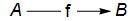• Warning: The notation “$f:A\to B$” is like that used for functions. This notation may be used in any category, but it does not imply that $f$ is a function or that $A$ and $B$ have elements.
• For an arrow $f:A\to B$, the notation “$\text{dom}(f)$” refers to $A$ and “$\text{cod}(f)$” refers to $B$.
• For a given category $\mathsf{C}$, the collection of all the arrows with domain $A$ and codomain $B$ may be denoted by
• “$\text{Hom}(A,B)$” or
• “$\text{Hom}_\mathsf{C}(A,B)$” or
• “$\mathsf{C}(A,B)$”.

• Some newer books and articles in category theory use the name source for domain and target for codomain. This usage has the advantage that a newcomer to category theory will be less likely to think of an arrow as a function.

## Axiom 3: Composition

If $f$ and $g$ are arrows in a category for which $\text{cod}(f)=\text{dom}(g)$, as in this diagram:then there is a unique arrow with domain $A$ and codomain $C$ called the composite of $f$ and $g$.

### Notes for Axiom 3

• The unique arrow required by Axiom 3 may be denoted by “$g\circ f$” or “$gf$”. “$g\circ f$” is more explicit, but “$gf$” is much more commonly used by category theorists.
• Many constructions in categories may be shown by diagrams, like the one used just above.
• The diagramis said to commute if $h=g\circ f$.

• It is useful to think of $f$ followed by $g$ as a path in the diagram. Then a metaphor for composition is: Every path of length 2 has exactly one composite.
• It is customary in some texts in category theory to indicate that a diagram commutes by putting a gyre in the middle:• Note that the composite of the path that I described as “$f$ followed by $g$” is written as “$g\circ f$” or “$gf$”, which seems backward. Nevertheless, the most common notation in category theory for the composite of “$f$ followed by $g$” is $gf$. Some authors in computer science write “$f;g$” for “$gf$” to get around this problem.
• The concept of category is an abstraction of the idea of function, and the composition of arrows is an abstraction of the composition of functions. It uses the same notation, “$g\circ f$”. If $f$ and $g$ are set functions, then for an element $x$ in the domain of $f$, $(g\circ f)(x)=g(f(x))$
• But in arbitrary category, it may make no sense to evaluate an arrow $f$ at some element $x$; indeed, the domain of $f$ may not have elements at all, and then the statement “$(g\circ f)(x)=g(f(x))$” is meaningless.

## Axiom 4: Identity arrows

Note: WordpPress does not recognize the html command

. Axiom 1 should be 4a, Axiom 2 4b Axiom 3 4c and Axiom 4 4d.

1. For each object $A$ of a category, there is an arrow denoted by $\mathsf{id}_A$.
2. $\textsf{dom}(\textsf{id}_A)=A$ and $\textsf{cod}(\textsf{id}_A)=A$.
3. For any object $B$ and any arrow $f:B\to A$, the diagram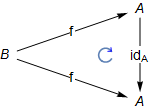commutes.

4. For any object $C$ and any arrow $g:A\to C$, the diagram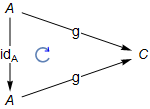commutes.

### Notes for Axiom 4

• The fact stated in Axiom 4(b) could be shown diagrammatically either asor as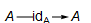• Facts (c) and (d) can be written in algebraic notation: For any arrow $f$ going to $A$,$\textsf{id}_A\circ f=f$and for any arrow $g$ coming from $A$,$g\circ \textsf{id}_A=g$
• There may be many arrows with domain and codomain both equal to $A$ (for example in the category $\mathsf{Set}$), but only one of them is $\textsf{id}_A$. It can be proved that $\textsf{id}_A$ is the unique arrow satisfying both (c) and (d) of the axiom.

## Axiom 5: Associativity

1. If $f$, $g$ and $h$ are arrows in a category for which $\text{cod}(f)=\text{dom}(g)$ and $\text{cod}(g)=\text{dom}(h)$, as in this diagram:then there is a unique arrow $k$ with domain $A$ and codomain $D$ called the composite of $f$, $g$ and $h$.

2. In the diagram below, the two triangles containing $k$ must both commute.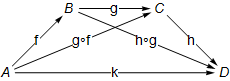### Notes for Axiom 5

• Axiom 5b requires that $h\circ(g\circ f)=(h\circ g)\circ f$(which both equal $k$), which is the usual algebraic notation for associativity.
• Note that the top two triangles commute by Axiom 3.
• The associativity axiom means that we can get rid of parentheses and write $k=h\circ f\circ g$just as we do for addition and multiplication of numbers.
• In my opinion the notation using categorical diagrams communicates information much more clearly than algebraic notation does. In particular, you don’t have to remember the domains and codomains of the functions — they appear in the picture. I admit that diagrams take up much more space, but now that we read math stuff on a computer screen instead of on paper, space is free.

# Examples of categories

For these examples, I give a detailed explanation about how they fit the definition of category.

## Example 1: MyFin

This first example is a small, finite category which I have named $\mathsf{MyFin}$ (“my finite category”). It is not at all an important category, but it has advantages as a first example.

• It’s small enough that you can see all the objects and arrows on the screen at once, but big enough not to be trivial.
• The objects and arrows have no properties other than being objects and arrows. (Some of the other examples involve familiar math objects.)
• So in order to check that $\mathsf{MyFin}$ really obeys the axioms for a category, you can use only the skeletal information given here. As a result, you must really understand the axioms!

A correct proof will be based on axioms and theorems.
The proof can be suggested by your intuitions,
but intuitions are not enough.
When working with $\mathsf{MyFin}$ you won’t have any intuitions!

### A diagram for $\mathsf{MyFin}$

This diagram gives a partial description of $\mathsf{MyFin}$.Now let’s see how to make the diagram above into a category.

### Axiom 1: Data

• The objects of $\mathsf{MyFin}$ are $A$, $B$, $C$ and $D$.
• The arrows are $f$, $g$, $h$, $j$, $k$, $r$, $s$, $u$, $v$, $w$ and $x$.
• You can regard the letters just listed as names of the objects and arrows. The point is that at this stage all you know about the objects and arrows are their names.
• If you prefer, you can think of the arrows as the actual arrows shown in the $\mathsf{MyFin}$ diagram.
• Our definition of $\mathsf{MyFin}$ is an abstract definition. You may have seen multiplication tables of groups given in terms of undefined letters. (If you haven’t, don’t worry.) Those are also abstract definitions.
• Our other definitions of categories involve math objects you actually know something about.

### Axiom 2: Domain and Codomain

• The domains and codomains of the arrows are shown by the diagram above.
• For example, $\text{dom}(r)=A$ and $\text{cod}(r)=C$, and $\text{dom}(v)=\text{cod}(v)=B$.

### Axiom 3: Composition

Showing the $\mathsf{MyFin}$ diagram does not completely define $\mathsf{MyFin}$. We must say what the composites of all the paths of length 2 are.

• In fact, most of them are forced, but two of them are not.
• We must have $g\circ f=r$ because $r$ is the only arrow possible for the composite, and Axiom 3 requires that every path of length 2 must have a composite.
• For the same reason, $h\circ g=s$.
• All the paths involving $u$, $v$, $w$ and $x$ are forced:

• (p1) $u\circ u=u$, $v\circ v=v$, $w\circ w=w$ and $x\circ x=x$.
• (p2) $f\circ u=f$, $r\circ u=r$, $j\circ u=j$ and $k\circ u=k$. You can see that, for example, $f\circ u=f$ by opening up the loop on $f$ like this: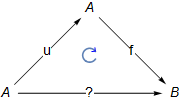There is only one arrow going from $A$ to $B$, namely$f$, so $f$ has to be the composite $f\circ u$.

• (p3) $v\circ f=f$, $g\circ v=g$ and $s\circ v=s$.
• (p4) $w\circ g=g$, $w\circ r=r$ and $h\circ w=h$.
• (p5) $x\circ h=h$, $x\circ s=s$, $x\circ j=j$ and $x\circ k=k$.
• For $s\circ f$ and $h\circ r$, we have to choose between $j$ and $k$ as composites. Since $s\circ f=(h\circ g)\circ f$ and $h\circ r=h\circ (g\circ f)$, Axiom 3 requires that we must chose one of $j$ and $k$ to be both composites.

Definition: $s\circ f=h\circ r=j$.

If we had defined $s\circ f=h\circ r=k$ we would have a different category, although one that is “isomorphic” to $\mathsf{MyFin}$ (you have to define “isomorphic” or look it up.)

### Axiom 5: Associativity

• Since we have already required both $(h\circ g)\circ f$ and $h\circ(g\circ f)$ to be $k$, composition is associative.

## Example 2: IntegerDiv

• This example uses familiar mathematical objects — positive integers.
• The arrows are not functions that can be applied to elements, since integers do not have elements.

### Axiom 1: Data

• The objects of IntegerDiv are all the positive integers.
• Suppose $m$ and $n$ are positive integers:
• If $m$ divides $n$, there is exactly one arrow from $m$ to $n$. I will call this arrow $\textsf{mdn}$. (This is my notation. There is no standard notation for this category.) For example there is one arrow from $2$ to $6$, denoted by $\textsf{2d6}$.
• If $m$ does not divide $n$, there is no arrow from $m$ to $n$.

### Axiom 2: Domain and codomain

The arrow denoted by $\textsf{mdn}$ has domain $m$ and codomain $n$.

##### Example##### Example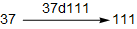##### Examplewhich may also be shown as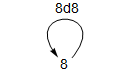### Axiom 3: Composition

The composite of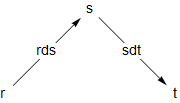must be $\textsf{rdt}$, since that is the only arrow with domain $r$ and codomain $t$.

This fact can also be written this way: $\mathsf{sdt}\circ\textsf{rds}=\textsf{rdt}$

### Axiom 4: Identity arrows

The composites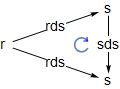andmust commute since the arrows shown are the only possible arrows with the domains and codomains shown. In other words, $\textsf{id}_\textsf{r}=\textsf{rdr}$ and $\textsf{id}_\textsf{s}=\textsf{sds}$.

### Axiom 5: Associativity

In the diagram below,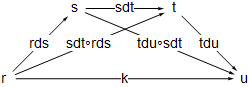there is only one arrow from one integer to another, so $\textsf{k}$ must be both $\textsf{tdu}\circ(\textsf{sdt}\circ\textsf{rds})$ and $(\textsf{tdu}\circ\textsf{sdt})\circ\textsf{rds}$ as required.

## Example 3: The category of Sets

In this section, I define the category $\mathsf{Set}$ (that is standard terminology in category theory.) This example will be very different from $\mathsf{MyFin}$, because it involves known mathematical objects — sets and functions.

### Axiom 1: Data

• Every set is an object of $\mathsf{Set}$ and nothing else is.
• Every function between sets is an arrow of $\mathsf{Set}$ and nothing else is an arrow of $\mathsf{Set}$.

### Axiom 2: Domain and codomain

For a given function $f$, $\text{dom}(f)$ is the domain of the function $f$ in the usual sense, and $\text{cod}(f)$ is the codomain of $f$ in the usual sense. (See Functions: specification and definition for more about domain and codomain.)

#### Examples

• Let $f:\mathbb{R}\to\mathbb{R}$ be the function defined by $f(x):=x^2$. Then the arrow $f$ in $\mathsf{Set}$ satisfies $\text{dom}(f)= \mathbb{R}$ and also $\text{cod}(f)=\mathbb{R}$.
• Let $j:\{1,2,3\}\to\{1,2,3,4\}$ be defined by $j(1):=1$, $j(2):=4$ and $j(3):=3$. Then $\text{dom}(j)=\{1,2,3\}$ and $\text{cod}(j)=\{1,2,3,4\}$.

### Axiom 3: Composition

The composite of $f:A\to B$ and $g:B\to C$ is the function $g\circ f:A\to C$ defined by $\text{(DC)}\,\,\,\,\,\,\,\,\,\,(g\circ f)(a):=g(f(a))$

#### Note

Many other categories have a similar definition of composition, including categories whose objects are math structures with underlying sets and whose arrows are structure-preserving functions between the underlying sets. But be warned: There are many useful categories whose arrows do not evaluate at an element of an object because the objects don’t have elements. In that case, (DC) is meaningless. This is true of $\mathsf{MyFin}$ and $\mathsf{IntegerDiv}$.

### Axiom 4: Identity arrows

For a set $A$, the identity arrow $\textsf{id}_A:A\to A$ is, as you might expect, the identity function defined by $\textsf{id}_A(a)=a$ for every $a\in A$. We must prove that these diagrams commute:The calculations below show that they commute. They use the definition of composite given by (DC).

• For any $b\in B$, $(\textsf{id}_A\circ f)(b)=\textsf{id}_A(f(b))=f(b)$
• For any $a\in A$, $(g\circ \textsf{id}_A)(a)=g(\textsf{id}_A(a))=g(a)$

Note: In $\mathsf{Set}$, there are generally many arrows from a particular set $S$ to itself (for example there are $4$ from $\{1,2\}$ to itself), but only one is the identity arrow.

### Axiom 5: Associativity

Composition of arrows in $\mathsf{Set}$ is associative because function composition is associative. Suppose we have functions as in this diagram:We must show that the two triangles containing $k$ in this diagram commute:In algebraic notation, this requires showing that for every element $a\in A$,$(h\circ(g\circ f))(a))=((h\circ g)\circ f)(a)$

The calculation below does that. It makes repeated use of Definition (DC) of composition. For any $a\in A$,$\begin{equation} \begin{split} \big(h\circ (g\circ f)\big)(a) & = h\big((g\circ f)(a)\big) \\ & = h\big(g(f(a))\big) \\ & = (h\circ g)(f(a)) \\ & = \big((h\circ g)\circ f\big)(a) \end{split} \end{equation}$

## Example 4: The category of Monoids

• This definition makes repeated use of the fact that a homomorphism of monoids is a set function. Specifically, if $(S,\Delta)$ and $(T,\nabla)$ are monoids with identities $e_S$ and $e_{T}$, a homomorphism $h:S\to T$ must be a set function that satisfies the following two axioms: $\text{(ME)}\,\,\,\,\,\,\,\,h(e_S)=e_T$ and for all elements $s, s’$ of $S$, $\text{(MM)}\,\,\,\,\,\,\,\,h(s\Delta s’)=h(s)\nabla h(s’)$
• The category of monoids may be denoted by $\mathsf{Mon}$.

### Axiom 1: Data

• Every monoid is an object of the category of monoids, and nothing else is.
• If $f$ is a homomorphism of monoids, then $f$ is an arrow of the category of monoids, and nothing else is.

### Axiom 2: Domain and codomain

If $(S,\Delta)$ and $(T,\nabla)$ are monoids and $f:(S,\Delta)\to(T,\nabla)$ is a homomorphism of monoids, then the domain of $f$ is $(S,\Delta)$ and the codomain of $f$ is $(T,\nabla)$.

#### Notes

• Since $f$ takes elements of the set $S$ to elements of the set $T$, it is also an arrow in the category $\mathsf{Set}$. In general, very few functions from $S$ to $T$ will be monoid homomorphisms from $(S,\Delta)$ to $(T,\nabla)$.
• Many authors do not distinguish between $f$ regarded as an arrow of $\mathsf{Mon}$ and $f$ regarded as an arrow of $\mathsf{Set}$. Others may write $U(f)$ for the arrow in $\mathsf{Set}$. “$U$” stands for “underlying functor“, also called “forgetful functor”.

### Axiom 3: Composition

The composite ofis the composite $g\circ f$ as set functions: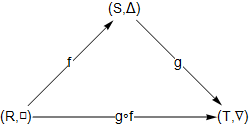It is necessary to check that $g\circ f$ is a monoid homomorphism. The following calculation shows that it preserves the monoid operation; it makes repeated use of equations (DC) and (MM).

The calculation: For elements $r$ and $r’$ of $R$,\begin{align*} (g\circ f)(r\,{\square}\, r’) &=g\left(f(r\, {\square}\, r’)\right)\,\,\,\,\,\text{(DC)}\\ &=g\left(f(r) {\scriptstyle\, \Delta}\, f(r’)\right)\,\,\,\,\,\text{(MM)}\\ &=g(f(r)){\,\nabla}\, g(f(r’))\,\,\,\,\text{(MM)}\\ &=(g\circ f)(r){\,\nabla}\,(g\circ f)(r’)\,\,\,\,\,\text{(DC)} \end{align*}

The fact that $g\circ f$ preserves the identity of the monoid is shown in the next section.

### Axiom 4: Identity arrows

For a monoid $(S,\Delta)$, the identity function $\text{id}_S:S\to S$ preserves the monoid operation $\Delta$, because $\text{id}_S(s\Delta s’)=s\Delta s’$ by definition of the identity function, and that is $\text{id}_S(s)\Delta \text{id}_S(s’)$ for the same reason.

The required diagrams below must commute because the set functions commute and, by Axiom 3, the set composition of a monoid homomorphism is a monoid homomorphism.We also need to show that $g\circ f$ as inpreserves identities. This calculation proves that it does; it uses (DC) and (ME)

\begin{align*} (g\circ f)(\text{id}_R) &=g(f((\text{id}_R))\\ &=g(\text{id}_S)\\ &=\text{id}_T \end{align*}

### Axiom 5: Associativity

The diagramin the category $\mathsf{Set}$ commutes, so the diagrammust also commute.

# References

All these references are available on line.Send to Kindle

# A new kind of introduction to category theory

This post has been replaced by the post A slow introduction to category theory.Send to Kindle

# Introducing abstract topics

I have been busy for the past several years revising abstractmath.org (abmath). Now I believe, perhaps foolishly, that most of the articles in abmath have reached beta, so now it is time for something new.

For some time I have been considering writing introductions to topics in abstract math, some typically studied by undergraduates and some taken by scientists and engineers. The topics I have in mind to do first include group theory and category theory.

The point of these introductions is to get the student started at the very beginning of the topic, when some students give up in total confusion. They meet and fall off of what I have called the abstraction cliff, which is discussed here and also in my blog posts Very early difficulties and Very early difficulties II.

I may have stolen the phrase “abstraction cliff” from someone else.

## Group theory

Group theory sets several traps for beginning students.

### Multiplication table

• A student may balk when a small finite group is defined using a set of letters in a multiplication table.
“But you didn’t say what the letters are or what the multiplication is?”
• Such a definition is an abstract definition, in contrast to the definition of “prime”, for example, which is stated in terms of already known entities, namely the integers.
• The multiplication table of a group tells you exactly what the binary operation is and any set with an operation that makes such a table correct is an example of the group being defined.
• A student who has no understanding of abstraction is going to be totally lost in this situation. It is quite possible that the professor has never even mentioned the concept of abstract definition. The professor is probably like most successful mathematicians: when they were students, they understood abstraction without having to have it explained, and possibly without even noticing they did so.

### Cosets

• Cosets are a real killer. Some students at this stage are nowhere near thinking of a set as an object or a thing. The concept of applying a binary operation on a pair of sets (or any other mathematical objects with internal structure) is completely foreign to them. Did anyone ever talk to them about mathematical objects?
• The consequence of this early difficulty is that such a student will find it hard to understand what a quotient group is, and that is one of the major concepts you get early in a group theory course.
• The conceptual problems with multiplication of cosets is similar to those with pointwise addition of functions. Given two functions $f,g:\mathbb{R}\to\mathbb{R}$, you define $f+g$ to be the function $(f+g)(x):=f(x)+g(x)$ Along with pointwise multiplication, this makes the space of functions $\mathbb{R}\to\mathbb{R}$ a ring with nice properties.
• But you have to understand that each element of the ring is a function thought of as a single math object. The values of the function are properties of the function, but they are not elements of the ring. (You can include the real numbers in the ring as constant functions, but don’t confuse me with facts.)
• Similarly the elements of the quotient group are math objects called cosets. They are not elements of the original group. (To add to the confusion, they are also blocks of a congruence.)

### Isomorphic groups

• Many books, and many professors (including me) regard two isomorphic groups as the same. I remember getting anguished questions: “But the elements of $\mathbb{Z}_2$ are equivalence classes and the elements of the group of permutations of $\{1,2\}$ are functions.”
• I admit that regarding two isomorphic groups as the same needs to be treated carefully when, unlike $\mathbb{Z}_2$, the group has a nontrivial automorphism group. ($\mathbb{Z}_3$ is “the same as itself” in two different ways.) But you don’t have to bring that up the first time you attack that subject, any more than you have to bring up the fact that the category of sets does not have a set of objects on the first day you define categories.

## Category theory

Category theory causes similar troubles. Beginning college math majors don’t usually meet it early. But category theory has begun to be used in other fields, so plenty of computer science students, people dealing with databases, and so on are suddenly trying to understand categories and failing to do so at the very start.

The G&G post A new kind of introduction to category theory constitutes an alpha draft of the first part of an article introducing category theory following the ideas of this post.

### Objects and arrows are abstract

• Every once in a while someone asks a question on Math StackExchange that shows they have no idea that an object of a category need not have elements and that morphisms need not be functions that take elements to elements.
• One questioner understood that the claim that a morphism need not be a function meant that it might be a multivalued function.

### Duality

• That misunderstanding comes up with duality. The definition of dual category requires turning the arrows around. Even if the original morphism takes elements to elements, the opposite morphism does not have to take elements to elements. In the case of the category of sets, an arrow in $\text{Set}^{op}$ cannot take elements to elements — for example, the opposite of the function $\emptyset\to\{1,2\}$.
• The fact that there is a concrete category equivalent to $\text{Set}^{op}$ is a red herring. It involves different sets: the function corresponding to the function just mentioned goes from a four-element set to a singleton. But in the category $\text{Set}^{op}$ as defined it is simply an arrow, not a function.

### Not understanding how to use definitions

• Some of the questioners on Math Stack Exchange ask how to prove a statement that is quite simple to prove directly from the definitions of the terms involved, but what they ask and what they are obviously trying to do is to gain an intuition in order to understand why the statement is true. This is backward — the first thing you should do is use the definition (at least in the first few days of a math class — after that you have to use theorems as well!
• I have discussed this in the blog post Insights into mathematical definitions (which gives references to other longer discussions by math ed people). See also the abmath section Rewrite according to the definitions.

## How an introduction to a math topic needs to be written

The following list shows some of the tactics I am thinking of using in the math topic introductions. It is quite likely that I will conclude that some tactics won’t work, and I am sure that tactics I haven’t mentioned here will be used.

• The introductions should not go very far into the subject. Instead, they should bring an exhaustive and explicit discussion of how to get into the very earliest part of the topic, perhaps the definition, some examples, and a few simple theorems. I doubt that a group theory student who hasn’t mastered abstraction and what proofs are about will ever be ready to learn the Sylow theorems.
• You can’t do examples and definitions simultaneously, but you can come close by going through an example step by step, checking each part of the definition.
• There is a real split between students who want the definitions first
(most of whom don’t have the abstraction problems I am trying to overcome)
and those who really really think they need examples first (the majority)
because they don’t understand abstraction.

• When you introduce an axiom, give an example of how you would prove that some binary operation satisfies the axiom. For example, if the axiom is that every element of a group must have an inverse, right then and there prove that addition on the integers satisfies the axiom and disprove that multiplication on integers satisies it.
• When the definition uses some undefined math objects, point out immediately with examples that you can’t have any intuition about them except what the axioms give you. (In contrast to definition of division of integers, where you and the student already have intuitions about the objects.)
• Make explicit the possible problems with abstractmath.org and Gyre&Gimble) will indeed find it difficult to become mathematical researchers — but not impossible!
• But that is not the point. All college math professors will get people who will go into theoretical computing science, and therefore need to understand category theory, or into particle physics, and need to understand groups, and so on.
• By being clear at the earliest stages of how mathematicians actually do math, they will produce more people in other fields who actually have some grasp of what is going on with the topics they have studied in math classes, and hopefully will be willing to go back and learn some more math if some type of math rears its head in the theories of their field.
• Besides, why do you want to alienate huge numbers of people from math, as our way of teaching in the past has done?
• “Our” means grammar school teachers, high school teachers and college professors.

### Acknowledgment

Thanks to Kevin Clift for corrections.Send to Kindle

# Representations of functions III

## Introduction to this post

I am writing a new abstractmath chapter called Representations of Functions. It will replace some of the material in the chapter Functions: Images, Metaphors and Representations. This post is a draft of the sections on representations of finite functions.

The diagrams in this post were created using the Mathematica Notebook Constructions for cographs and endographs of finite functions.nb.
You can access this notebook if you have Mathematica, which can be bought, but is available for free for faculty and students at many universities, or with Mathematica CDF Player, which is free for anyone and runs on Windows, Mac and Linux.

Like everything in abstractmath.org, the notebooks are covered by a Creative Commons ShareAlike 3.0 License.

## Graphs of finite functions

When a function is continuous, its graph shows up as a curve in the plane or as a curve or surface in 3D space. When a function is defined on a set without any notion of continuity (for example a finite set), the graph is just a set of ordered pairs and does not tell you much.

A finite function $f:S\to T$ may be represented in these ways:

• Its graph $\{(s,f(s))|s\in S\}$. This is graph as a mathematical object, not as a drawing or as a directed graph — see graph (two meanings)).
• A table, rule or two-line notation. (All three of these are based on the same idea, but differ in presentation and are used in different mathematical specialties.)
• By using labels with arrows between them, arranged in one of two ways:
• A cograph, in which the domain and the codomain are listed separately.
• An endograph, in which the elements of the domain and the codomain are all listed together without repetition.

All these techniques can also be used to show finite portions of infinite discrete functions, but that possibility will not be discussed here.

### Introductory Example

Let $\text{f}:\{a,b,c,d,e\}\to\{a,b,c,d\}$ be the function defined by requiring that $f(a)=c$, $f(b)=a$, $f(c)=c$, $f(d)=b$, and $f(e)=d$.

#### Graph

The graph of $f$ is the set
$(a,c),(b,a),(c,c),(d,b),(e,d)$
As with any set, the order in which the pairs are listed is irrelevant. Also, the letters $a$, $b$, $c$, $d$ and $e$ are merely letters. They are not variables.

#### Table

$\text{f}$ is given by this table:This sort of table is the format used in databases. For example, a table in a database might show the department each employee of a company works in:#### Rule

The rule determined by the finite function $f$ has the form

$(a\mapsto b,b\mapsto a,c\mapsto c,d\mapsto b,e\mapsto d)$

Rules are built in to Mathematica and are useful in many situations. In particular, the endographs in this article are created using rules. In Mathematica, however, rules are written like this:

$(a\to b,b\to a,c\to c,d\to b,e\to d)$

This is inconsistent with the usual math usage (see barred arrow notation) but on the other hand is easier to enter in Mathematica.

In fact, Mathematica uses very short arrows in their notation for rules, shorter than the ones used for the arrow notation for functions. Those extra short arrows don’t seems to exist in TeX.

#### Two-line notation

Two-line notation is a kind of horizontal table.

$\begin{pmatrix} a&b&c&d&e\\c&a&c&b&d\end{pmatrix}$

The three notations table, rule and two-line do the same thing: If $n$ is in the domain, $f(n)$ is shown adjacent to $n$ — to its right for the table and the rule and below it for the two-line.

Note that in contrast to the table, rule and two-line notation, in a cograph each element of the codomain is shown only once, even if the function is not injective.

#### Cograph

To make the cograph of a finite function, you list the domain and codomain in separate parallel rows or columns (even if the domain and codomain are the same set), and draw an arrow from each $n$ in the domain to $f(n)$ in the codomain.

This is the cograph for $\text{f}$, represented in columns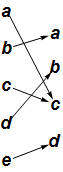and in rows (note that $c$ occurs only once in the codomain)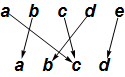Pretty ugly, but the cograph for finite functions does have its uses, as for example in the Wikipedia article composition of functions.

In both the two-line notation and in cographs displayed vertically, the function goes down from the domain to the codomain. I guess functions obey the law of gravity.

##### Rearrange the cograph

There is no expectation that in the cograph $f(n)$ will be adjacent to $n$. But in most cases you can rearrange both the domain and the codomain so that some of the structure of the function is made clearer; for example: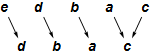The domain and codomain of a finite function can be rearranged in any way you want because finite functions are not continuous functions. This means that the locations of points $x_1$ and $x_2$ have nothing to do with the locations of $f(x_1)$ and $f(x_2)$: The domain and codomain are discrete.

#### Endograph

The endograph of a function $f:S\to T$ contains one node labeled $s$ for each $s\in S\cup T$, and an arrow from $s$ to $s’$ if $f(s)=s’$. Below is the endograph for $\text{f}$.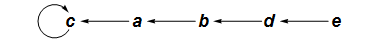The endograph shows you immediately that $\text{f}$ is not a permutation. You can also see that with whatever letter you start with, you will end up at $c$ and continue looping at $c$ forever. You could have figured this out from the cograph (especially the rearranged cograph above), but it is not immediately obvious in the cograph the way it in the endograph.

There are more examples of endographs below and in the blog post
A tiny step towards killing string-based math. Calculus-type functions can also be shown using endographs and cographs: See Mapping Diagrams from A(lgebra) B(asics) to C(alculus) and D(ifferential) E(quation)s, by Martin Flashman, and my blog posts Endographs and cographs of real functions and Demos for graph and cograph of calculus functions.

### Example: A permutation

Suppose $p$ is the permutation of the set $\{0,1,2,3,4,5,6,7,8,9\}$given in two-line form by
$\begin{pmatrix} 0&1&2&3&4&5&6&7&8&9\\0&2&1&4&5&3&7&8&9&6\end{pmatrix}$

#### Cograph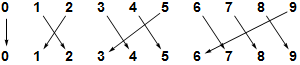#### Endograph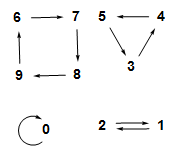Again, the endograph shows the structure of the function much more clearly than the cograph does.

The endograph consists of four separate parts (called components) not connected with each other. Each part shows that repeated application of the function runs around a kind of loop; such a thing is called a cycle. Every permutation of a finite set consists of disjoint cycles as in this example.

#### Disjoint cycle notation

Any permutation of a finite set can be represented in disjoint cycle notation: The function $p$ is represented by:

$(0)(1,2)(3,4,5)(6,7,8,9)$

Given the disjoint cycle notation, the function can be determined as follows: For a given entry $n$, $p(n)$ is the next entry in the notation, if there is a next entry (instead of a parenthesis). If there is not a next entry, $p(n)$ is the first entry in the cycle that $n$ is in. For example, $p(7)=8$ because $8$ is the next entry after $7$, but $p(5)=3$ because the next symbol after $5$ is a parenthesis and $3$ is the first entry in the same cycle.

The disjoint cycle notation is not unique for a given permutation. All the following notations determine the same function $p$:

$(0)(1,2)(4,5,3)(6,7,8,9)$
$(0)(1,2)(8,9,6,7)(3,4,5)$
$(1,2)(3,4,5)(0)(6,7,8,9)$
$(2,1)(5,3,4)(9,6,7,8)$
$(5,3,4)(1,2)(6,7,8,9)$

Cycles such as $(0)$ that contain only one element are usually omitted in this notation.

### Example: A tree

Below is the endograph of a function $t:\{0,1,2,3,4,5,6,7,8,9\}\to\{0,1,2,3,4,5,6,7,8,9\}$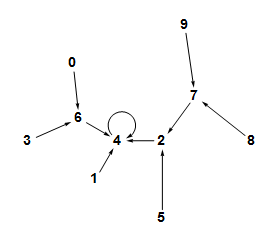This endograph is a tree. The graph of a function $f$ is a tree if the domain has a particular element $r$ called the root with the properties that

• $f(r)=r$, and
• starting at any element of the domain, repreatedly applying $f$ eventually produces $r$.

In the case of $t$, the root is $4$. Note that $t(4)=4$, $t(t(7))=4$, $t(t(t(9)))=4$, $t(1)=4$, and so on.

The endographshown here is also a tree.

See the Wikipedia article on trees for the usual definition of tree as a special kind of graph. For reading this article, the definition given in the previous paragraph is sufficient.

### The general form of a finite function

This is the endograph of a function $t$ on a $17$-element set:It has two components. The upper one contains one $2$-cycle, and no matter where you start in that component, when you apply $t$ over and over you wind up flipping back and forth in the $2$-cycle forever. The lower component has a $3$-cycle with a similar property.

This illustrates a general fact about finite functions:

• The endograph of any finite function contains one or more components $C_1$ through $C_k$.
• Each component $C_k$ contains exactly one $n_k$ cycle, for some integer $n_k\geq 1$, to which are attached zero or more trees.
• Each tree in $C_k$ is attached in such a way that its root is on the unique cycle contained in $C_k$.

In the example above, the top component has three trees attached to it, two to $3$ and one to $4$. (This tree does not illustrate the fact that an element of one of the cycles does not have to have any trees attached to it).

You can check your understanding of finite functions by thinking about the following two theorems:

• A permutation is a finite function with the property that its cycles have no trees attached to them.
• A tree is a finite function that has exactly one component whose cycle is a $1$-cycle.Send to Kindle

# Representations of functions II

## Introduction to this post

I am writing a new abstractmath chapter called Representations of Functions. It will replace some of the material in the chapter Functions: Images, Metaphors and Representations.

This post includes a draft of the introduction to the entire new chapter (immediately below) and of the sections on graphs of continuous functions of one variable with values in the plane and in 3-space. Later posts will concern multivariable continuous functions and finite discrete functions.

## Introduction to the new Chapter

Functions can be represented visually in many different ways. There is a sharp difference between representing continuous functions and representing discrete functions.

For a continuous function $f$, $f(x)$ and $f(x’)$ tend to be close together when $x$ and $x’$ are close together. That means you can represent the values at an infinite number of points by exhibiting them for a bunch of close-together points. Your brain will automatically interpret the points nearby that are not represented.

Nothing like this works for discrete functions. Many different arrangements of the inputs and outputs can be made. Different arrangements may be useful for representing different properties of the function.

### Illustrations

The illustrations were created using these Mathematica Notebooks:

These notebooks contain many more examples of the ways functions can be represented than are given in this article. The notebooks also contain some manipulable diagrams which may help you understand the diagrams. In addition, all the 3D diagrams can be rotated using the cursor to get different viewpoints. You can access these tools if you have Mathematica, which is available for free for faculty and students at many universities, or with Mathematica CDF Player, which runs on Windows, Mac and Linux.

Like everything in abstractmath.org, the notebooks are covered by a Creative Commons ShareAlike 3.0 License.

## Functions from a subset of $\mathbb{R}$ to $\mathbb{R}\times\mathbb{R}$

Suppose $F:\mathbb{R}\to\mathbb{R}\times\mathbb{R}$. That means you put in one number and get out a pair of numbers.

### The unit circle

An example is the unit circle, which is the graph of the function $t\mapsto(\cos t,\sin t)$. That has this parametric plot:Because $\cos^2 t+\sin^2 t=1$, every real number $t$ produces a point on the unit circle. Four point are shown. For example,$(\cos\pi,\,\sin\pi)=(-1,0)$ and
$(\cos(5\pi/3),\,\sin(5\pi/3))=(\frac{1}{2},\frac{\sqrt3}{2})\approx(.5,.866)$

#### $t$ as time

In graphing functions $f:\mathbb{R}\to\mathbb{R}$, the plot is in two dimensions and consists of the points $(x,f(x))$: the input and the output. The parametric plot shown above for $t\mapsto(\cos^2 t+\sin^2)$ shows only the output points $(\cos t,\sin t)$; $t$ is not plotted on the graph at all. So the graph is in the plane instead of in three-dimensional space.

An alternative is to use time as the third dimension: If you start at some number $t$ on the real line and continually increase it, the value $f(t)$ moves around the circle counterclockwise, repeating every $2\pi$ times. If you decrease $t$, the value moves clockwise. The animated gif circlemovie.gif shows how the location of a point on the circle moves around the circle as $t$ changes from $0$ to $2\pi$. Every point is traversed an infinite number of times as $t$ runs through all the real numbers.

#### The unit circle with $t$ made explicit

Since we have access to three dimensions, we can show the input $t$ explicitly by using a three-dimensional graph, shown below. The blue circle is the function $t\mapsto(\cos t,\sin t,0)$ and the gold helix is the function $t\mapsto(\cos t,\sin t,.2t)$.The introduction of $t$ as the value in the vertical direction changes the circle into a helix. The animated .gif covermovie.gif shows both the travel of a point on the circle and the corresponding point on the helix.

As $t$ changes, the circle is drawn over and over with a period of $2\pi$. Every point on the circle is traversed an infinite number of times as $t$ runs through all the real numbers. But each point on the helix is traversed exactly once. For a given value of $t$, the point on the helix is always directly above or below the point on the circle.

The helix is called the universal covering space of the circle, and the set of points on the helix over (and under) a particular point $p$ on the circle is called the fiber over $p$. The universal cover of a space is a big deal in topology.

### Figure-8 graph

This is the parametric graph of the function $t\mapsto(\cos t,\sin 2t)$.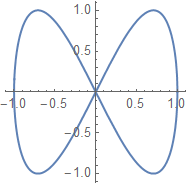Notice that it crosses itself at the origin, when $t$ is any odd multiple of $\frac{\pi}{2}$.

Below is the universal cover of the Figure-8 graph. As you can see, the different instances of crossing at $(0,0)$ are separated. The animated.gif Fig8movie shows the paths taken as $t$ changes on the figure 8 graph and on its universal cover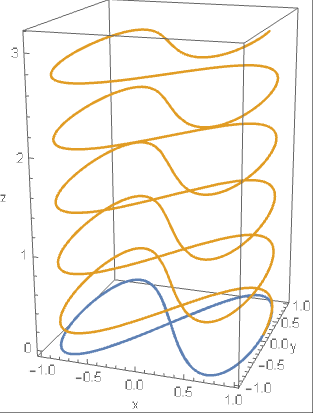## Functions from a subset of $\mathbb{R}$ to $\mathbb{R}\times\mathbb{R}\times\mathbb{R}$

The graph of a function from a subset of $\mathbb{R}$ to $\mathbb{R}\times\mathbb{R}\times\mathbb{R}$ can also be drawn as a parametric graph in three-dimensional space, giving a three-dimensional curve. The trick that I used in the previous section of showing the input parameter so that you can see the universal cover won’t work in this case because it would require four dimensions.

### Universal covers

The gold curves in the figures for the universal covers of the circle and the figure 8 are examples of functions from $\mathbb{R}$ to $\mathbb{R}\times\mathbb{R}\times\mathbb{R}$.

### The seven-pointed crown

Here are views from three different angles of the graph of the function $t\mapsto(\cos t, \sin t, \sin 7t)$: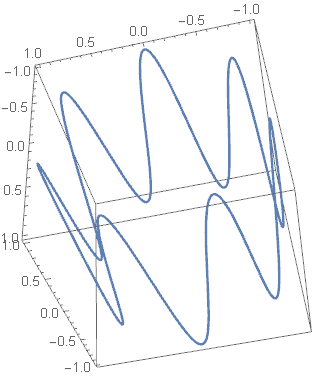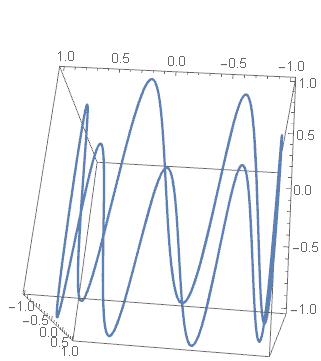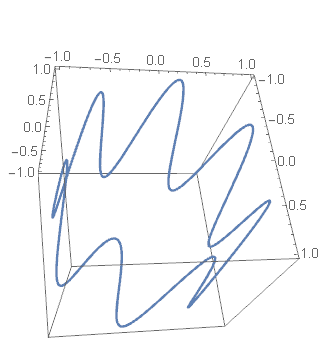The animated gif crownmovie.gif represents the parameter $t$ in time.

### Another curve in space

Below are two views of the curve defined by $t\mapsto({-4t^2+53t)/18,t,.4(-t^2+1-10t)}$.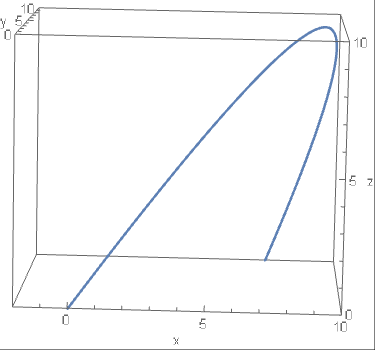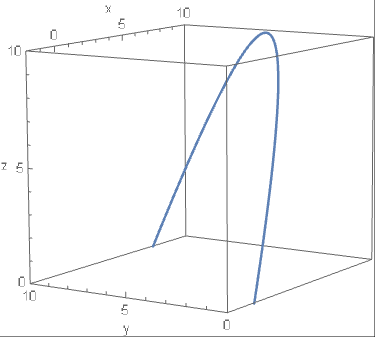The following plots the $x$-curve $-4t^2+53t)/18$ gold in the $yz$ plane and the $z$ curve $.4(-t^2+1-10t)$ in the $xy$ plane. The first and third views are arranged so that you see the curve just behind one of those two planes.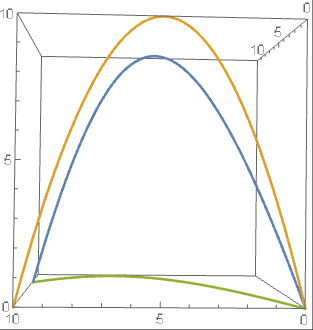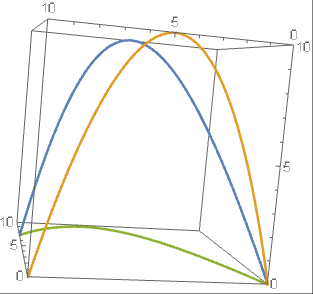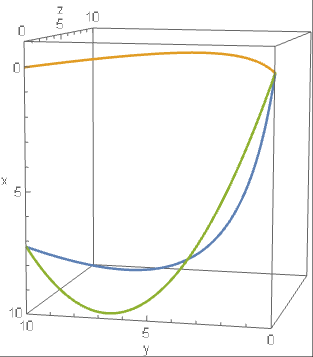Send to Kindle

# Representations of functions I

## Introduction to this post

I am writing a new abstractmath chapter called Representations of Functions. It will replace some of the material in the chapter Functions: Images, Metaphors and Representations.

This post includes a draft of the introduction to the new chapter (immediately below) and of the section Graphs of continous functions of one variable. Later posts will concern multivariable continuous functions, probably in two or three sections, and finite discrete functions.

## Introduction to the new abstractmath chapter on representations of functions

Functions can be represented visually in many different ways. There is a sharp difference between representing continuous functions and representing discrete functions.

For a continuous function $f$, $f(x)$ and $f(x’)$ tend to be close together when $x$ and $x’$ are close together. That means you can represent the values at an infinite number of points by exhibiting them for a bunch of close-together points. Your brain will automatically interpret the points nearby that are not represented.

Nothing like this works for discrete functions. As you will see in the section on discrete functions, many different arrangements of the inputs and outputs can be made. In fact, different arrangements may be useful for representing different properties of the function.

### Illustrations

The illustrations were created using these Mathematica Notebooks:

These notebooks contain many more examples of the ways functions can be represented than are given in this article. The notebooks also contain some manipulable diagrams which may help you understand the diagrams. In addition, all the 3D diagrams can be rotated using the cursor to get different viewpoints. You can access these tools if you have Mathematica, which is available for free for faculty and students at many universities, or with Mathematica CDF Player, which runs on Windows, Mac and Linux.

Like everything in abstractmath.org, the notebooks are covered by a Creative Commons ShareAlike 3.0 License.

## Graphs of continous functions of one variable

The most familiar representations of continuous functions are graphs of functions with one real variable. Students usually first see these in secondary school. Such representations are part of the subject called Analytic Geometry. This section gives examples of such functions.

There are other ways to represent continuous functions, in particular the cograph and the endograph. These will be the subject of a separate post.

The graph of a function $f:S\to T$ is the set of ordered pairs $\{(x,f(x))\,|\,x\in S\}$. (More about this definition here.)

In this section, I consider continuous functions for which $S$ and $T$ are both subsets of the real numbers. The mathematical graph of such a function are shown by plotting the ordered pairs $(x,f(x))$ as points in the two-dimensional $xy$-plane. Because the function is continuous, when $x$ and $x’$ are close to each other, $f(x)$ and $f(x’)$ tend to be close to each other. That means that the points that have been plotted cause your brain to merge together into a nice curve that allows you to visualize how $f$ behaves.

#### Example

This is a representation of the graph of the curve $g(x):=2-x^2$ for approximately the interval $(-2,2)$. The blue curve represents the graph.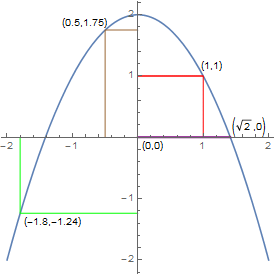The brown right-angled line in the upper left side, for example, shows how the value of independent variable $x$ at $(0.5)$ is plotted on the horizontal axis, and the value of $g(0.5)$, which is $1.75$, is plotted on the vertical axis. So the blue graph contains the point $(0.5,g(0.5))=(0.5,1.75)$. The animated gif upparmovie.gif shows a moving version of how the curve is plotted.

#### Fine points

• The mathematical definition of the graph is that it is the set $\{(x,2-x^2)\,|\,x\in\mathbb{R}\}$. The blue curve is not, of course, the mathematical graph, it represents the mathematical graph.
• The blue curve consists of a large but finite collection of pixels on your screen, which are close enough together to appear to form a continuous curve which approximates the mathematical graph of the function.
• Notice that I called the example the “representation of the graph” instead of just “graph”. That maintains the distinction between the mathematical ordered pairs $(x,g(x))$ and the pixels you see on the screen. But in fact mathe­maticians and students nearly always refer to the blue line of pixels as the graph. That is like pointing to a picture of your grandmother and saying “this is my grandmother”. There is nothing wrong with saying things that way. But it is worth understanding that two different ideas are being merged.

### Discontinuous functions

A discontinuous function which is continuous except for a small finite number of breaks can also be represented with a graph.

#### Example

Below is the function $f:\mathbb{R}\to\mathbb{R}$ defined by
f(x):=\left\{ \begin{align} 2-x^2\,\,\,\,\,\,\,\,\,\,\,\,\,\,\,\,\,\,\,\,\,\,(x\gt0) \\ 1-x^2\,\,\,\,\,\,(-1\lt x\lt 0) \\ 2-x^2\,\,\,\,\,\,\,\,\,\,\,\,\,\,\,\,\,(x\lt-1) \end{align}\right.##### Example

The Dirichlet function is defined by
$F(x):= \begin{cases} 1 & \text{if }x\text{ is rational}\\ \frac{1}{2} & \text{if }x\text{ is irrational}\\ \end{cases}$  for all real $x$.

The abmath article Examples of functions spells out in detail what happens when you try to draw this function.

### Graphs can fool you

The graph of a continuous function cannot usually show the whole graph, unless it is defined only on a finite interval. This can lead you to jump to conclusions.

##### Example

For example, you can’t tell from the the graph of the function $y=2-x^2$ whether it has a local minimum (because the graph does not show all of the function), although you can tell by using calculus on the formula that it does not have one. The graph looks like it might have a vertical asymptotes, but it doesn’t, again as you can tell from the formula.

by looking at its graph
is useful but dangerous.

##### Example

Below is the graph of the function
$f(x)=.0002{{\left( \frac{{{x}^{3}}-10}{3{{e}^{-x}}+1} \right)}^{6}}$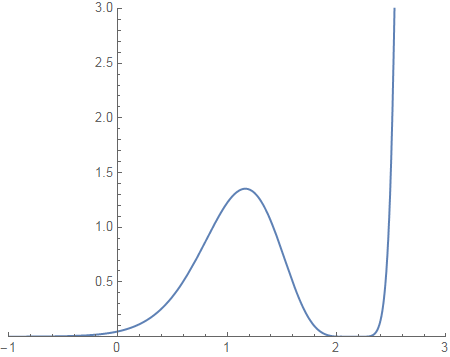If you didn’t know the formula for the function (but know it is continuous), you could still see that it has a local maximum somewhere to the right of $x=1$. It looks like it has one or more zeroes around $x=-1$ and $x=2$. And it looks like it has an asymptote somewhere to the right of $x=2.5$.

If you do know the formula, you can find out many things about the function that you can’t depend on the graph to see.

• You can see immediately that $f$ has a zero at $x=\sqrt{10}$, which is about $2.15$.
• If you notice that the denominator is positive for all $x$, you can figure out that
• $\sqrt{10}$ is the only root.
• $f(x)\geq0$ for all $x$.
• $f$ has an asymptote as $x\to-\infty$ (use L’Hôpital).
• Numerical analysis (I used Mathematica) shows that $f'(x)$ has two zeros, at $\sqrt{10}$ and at about $x=1.1648$. $f”(1.1648)$ is about $-10.67$ , which strongly suggests that $f$ has a local max near $1.1648$, consistent with the graph.
• Since $f$ is defined for every real number, it can’t have a vertical asymptote anywhere. The graph looks like it becomes vertical somewhere to the right of $x=2.4$, but that is simply an illustration of the unbelievably fast growth of any exponential function.
• The section on Zooming and Chunking gives other details.

## Acknowledgments

Sue VanHattum.Send to Kindle